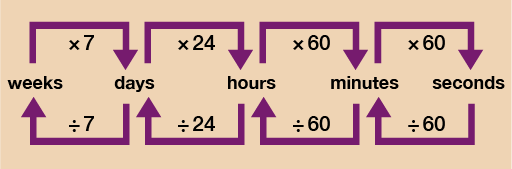Everyday maths 2

Start this free course now. Just create an account and sign in. Enrol and complete the course for a free statement of participation or digital badge if available.

Free course

# 3.2 Converting units of time

You can see from the diagram below that to convert units of time you can use a very similar method to the one you used when converting other units of measure. There is one slight difference when working with time however.Figure 17 A conversion chart for time

Let’s say you want to work out how long 245 minutes is in hours. The diagram above shows that you should do 245 ÷ 60 = 4.083. This is not a particularly helpful answer since you really want the answer in the format of: ___ hours __ minutes. Due to the fact that time does not work in 10s, you need to do a little more work once arriving at your answer of 4.083.

The answer is obviously 4 hours and an amount of minutes.

4 hours then is 4 × 60 = 240 minutes.

Since you wanted to know how long 245 minutes is you just do 245 – 240 = 5 minutes left over. So 245 minutes is 4 hours and 5 minutes.

It’s a very similar process if you want to go from say minutes to seconds. Let’s take it you want to know how long 5 minutes and 17 seconds is in seconds. 5 minutes would be 5 × 60 = 300 seconds. You then have a further 17 seconds to add on so you do 300 + 17 = 317 seconds.

Have a go at the activity below to make sure you feel confident with converting times.

## Activity 6: Converting times

Convert the following times:

• a.6 hours and 35 minutes = ___ minutes.
• b.85 minutes = ____ hours and ____ minutes.
• c.153 seconds = ____ minutes and ___ seconds.
• d.46 days = ___ weeks and ____days.
• e.3 minutes and 40 seconds = ____ seconds.

### Answer

• a.6 hours = 6 × 60 = 360 minutes

360 minutes + 35 minutes = 395 minutes

• b.85 minutes ÷ 60 = 1.416

1 hour = 60 minutes.

85 minutes − 60 minutes = 25 minutes remaining

So 85 minutes = 1 hour and 25 minutes

• c.153 seconds ÷ 60 = 2.55

2 minutes = 2 × 60 = 120 seconds

153 seconds − 120 seconds = 33 seconds remaining

So 153 seconds = 2 minutes and 33 seconds

• d.46 days ÷ 7 = 6.571…

6 weeks = 6 × 7 = 42 days

46 days − 42 days = 4 days remaining

So 46 days = 6 weeks and 4 days

• e.3 minutes = 3 × 60 = 180 seconds

180 seconds + 40 seconds = 220 seconds

Hopefully you found that activity fairly straightforward and are now feeling ready to move on to the last part of the ‘Units of measure’ session – Average speed.

FSM_2

### Take your learning further

Making the decision to study can be a big step, which is why you'll want a trusted University. The Open University has 50 years’ experience delivering flexible learning and 170,000 students are studying with us right now. Take a look at all Open University courses.

If you are new to University-level study, we offer two introductory routes to our qualifications. You could either choose to start with an Access module, or a module which allows you to count your previous learning towards an Open University qualification. Read our guide on Where to take your learning next for more information.

Not ready for formal University study? Then browse over 1000 free courses on OpenLearn and sign up to our newsletter to hear about new free courses as they are released.

Every year, thousands of students decide to study with The Open University. With over 120 qualifications, we’ve got the right course for you.

Request an Open University prospectus371